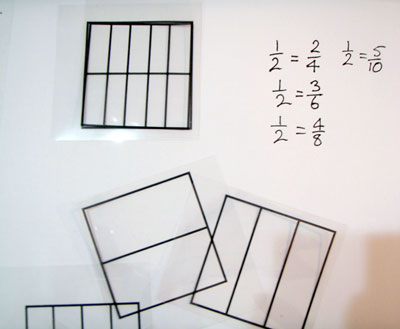# Overlay grids

It is important that students experience the decision-making process of selecting grids for themselves, and physically positioning them to make the new fractions.

Watch the video Using Overlay Grids.

Photocopy the overlay templates onto transparencies and make a set for each pair of students.

The grids can be used for a number of tasks.

• Find and record equivalent fractions for $$\frac{1}{2}$$, $$\frac{1}{3}$$, $$\frac{1}{5}$$ etc.
• Make a grid and record all the equivalent fractions (e.g. $$\frac{1}{3}$$ = $$\frac{2}{6}$$, $$\frac{2}{3}$$ = $$\frac{4}{6}$$, $$\frac{3}{3}$$ = $$\frac{6}{6}$$).
• Explore different ways to represent twelfths and record the equivalent fractions.Use of overlay templates.

Encourage students to look for patterns and relationships among the groups of equivalent fractions they find, particularly multiplicative relationships.

As students become familiar with finding equivalent fractions, ask them to predict what they will find (e.g. if looking for fractions equivalent to $$\frac{2}{3}$$). Students should explain their prediction strategies.